# CLASS 11 MATHS CHAPTER-5 COMPLEX NUMBER AND QUADRATIC EQUATIONS

### Exercise 5.1

Express each of the complex numbers given in the exercises 1 to 10 in the form a + ib:

Question1. Express each of the complex number in the form a + ib.

(5i)(−3/5i)

Solution :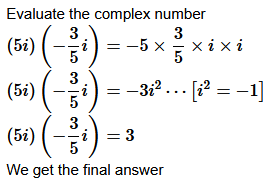Question2. Express each of the complex number in the form a + ib.

i9+i i19

Solution :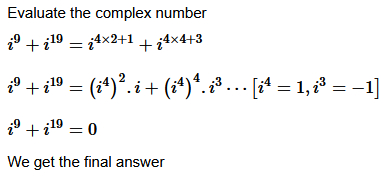Question3. Express each of the complex number in the form a + ib.

i−39

Solution :
Evaluate the complex number

i−39 = i4×9−3

i−39=(i4)−9.i−3

i−39=i⋯[i=−1]

i−39=i

Question4. Express each of the complex number in the form a + ib.

3(7+i7)+i(7+i7)

Solution :
Here Evaluate the complex number

3(7+i7)+i(7+i7)=21+21i+7i+7i2

3(7+i7)+i(7+i7)=21+28i+7i2⋯[i2=−1]

3(7+i7)+i(7+i7)=14+28i

Question5. Express each of the complex number in the form a + ib.

(1−i)−(−1+i6)

Solution :
Here Evaluate the complex number

(1−i) − (−1+6i) = 1−i+1 − i6

(1−i)−(−1+6i) = 2−7i

Question6.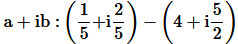Solution :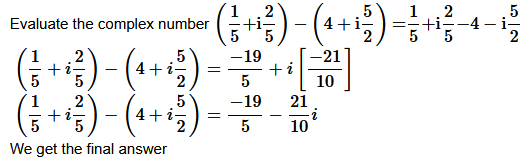Question7.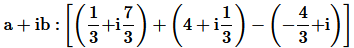Solution :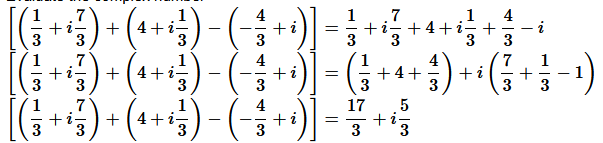we get the answear

Question8. Express each of the complex number in the form a + ib.

(1−i)4

Ans. Here Evaluate the complex number

(1−i)4=[1+i2−2i]2

(1−i)4=[1−1−2i]2

(1−i)=(−2i)×(−2i)
(1−i)=−4

Question 9.Express each of the complex number in the form a + ib.

(1/3+3i)3

Solution :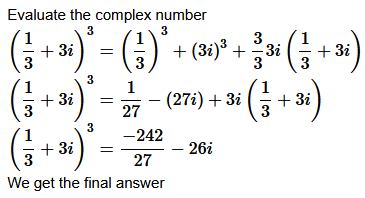Question10. Express each of the complex number in the form a + ib.

(−2−1/3 i)3

Solution :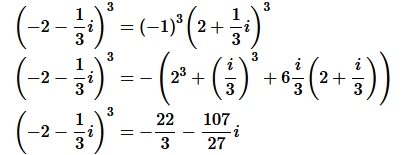Find the multiplicative inverse of each of the complex numbers given in the exercises 11 to 13.

Question11. Find the multiplicative inverse of each of the complex numbers given

4−3i

Solution :

Question12. Find the multiplicative inverse of the complex number √5 + 3i

Question13. Find the multiplicative inverse of each of the complex numbers given

−i

Solution :
Multiplicative Inverse of Let  z = −i
Then,
z¯=i  and   | z | = 1=1
Therefore, the multiplicative inverse of  −i is given by z−1 = z¯ | z| 2=i/1=i

Question14. Express the following expression in the form of a + ib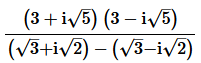Solution :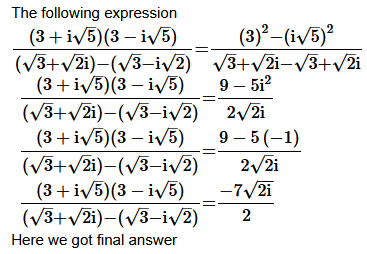### Exercise 5.2

Find the modulus and the argument of each of the complex numbers in exercises 1 to 2.

Question1. z = – 1 – i√3

Solution :
Given:

The complex number is

z = – 1 – i√3

Let  rcosθ  = -1   and   rsin θ = –√3

(rcosθ )2+(rsinθ )2=(−1)+ (–√3)2

r2(cos2θ +sin2θ )=1+3r2 = 4[cos2θ +sin2θ =1]

r= √4 = 2[   Conventionally,   r]
Modulus  =2

2cosθ =-1   and   2sin θ = –√3cosθ =−12  and   sin θ =–√3/2
Since both the values of  sinθ   and   cos θ

Question2.

z = -√3 + i

Solution :
Given: Let  rcosθ =–√3 and   rsin θ =1

(rcosθ )2+(rsinθ )2=(–√3)2+(−1)2

r2=3+1=4 [cos2θ +sin2θ =1]r = √ 4=2LLL[   Conventionally,   r0 ]

Modulus  =22cosθ = –√ 3and   2sin θ =1

cosθ =−√3/2  and   sin θ =12θ = π -π /6=5π/ 6LL[ As   θ   lies in the II quadrant ]

Thus, the modulus and argument of the complex number  −3–√+i  are   2   and   5π 6

Respectively,

Question3. Convert each of the complex numbers given in the polar form:

1−i

Solution :
Given: The complex number is
1−i

Let  rcosθ = 1   and   rsinθ = −1

r2cos2θ+r2sin2θ=12+(−1)2⇒r2(cos2θ+sin2θ)=1+1

r2=2/r= √2[   Conventionally,   r>0]

√2cosθ  = 1   and   √2sinθ =-1cosθ  = 1/√2  and   sin θ =-1/√2

θ =-π/ 4[   As   θ   liesin the IV quadrant   ]

1-i=rcos θ +irsin θ = √2 cos(−π /4) + i√2sin(−π /4 ) = √2 [cos(−π/4)+isin(−π /4)]

Required polar form

Question4. Convert each of the complex numbers given in the polar form:

−1+i

Solution :The complex number is

−1+i

Let  rcosθ =-1   and   rsin θ =1

r2cos2θ +r2sin2θ =(-1)2+12r2(cos2θ +sin2θ )=1+1r2=2r=  √2

2–√cosθ =-1   and   2–√sinθ =1

cosθ = -√12  and   √2sinθ = 1 /θ = π -π/ 4=3π /4L[As  θ   lies in the II quadrant]

It can be written,

-1+i=rcos θ +irsin θ =2–√cos3π 4+i  √2sin3π 4 =  √2(cos3π 4+isin3π / 4)

Required polar form

Question5. Convert each of the complex numbers given in the polar form:

−1−i

Solution :The complex number is

−1−i

Let  rcosθ =-1   and   rsin θ =-1

r2cos2θ +r2sin2θ =(-1)2+(−1)2r2(cos2θ +sin2θ )=1+1

r2=2

r=  √2

√2cosθ =-1   and   √2sinθ =-1

cosθ = -1/√2  and   sin θ =-1/√2

θ -(π – π /4)−3π/ 4  [As  0   lies in the III quadrant]

-1-i = rcos θ +irsin θ = √2cos−3π / 4 + i √2 sin − 3π / 4 = –√2(cos− 3π/ 4 + isin−3π/ 4)

Required polar form

Question6. Convert each of the complex numbers given in the polar form:

−3

Solution :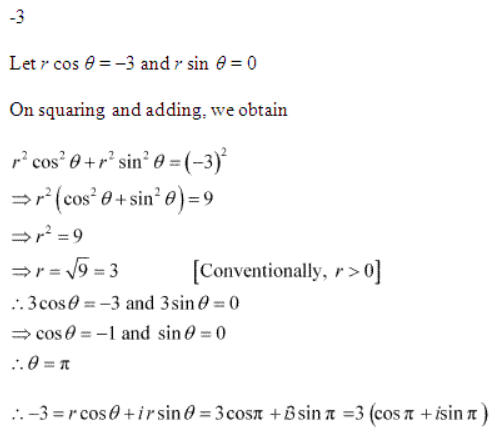Question7. Convert the given complex number in polar form √3 + i3–√+i

Solution :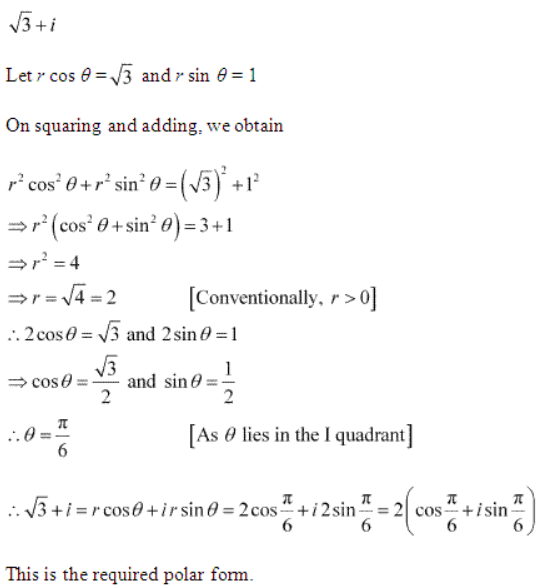Question8. Convert each of the complex numbers given in the polar form:

i

Solution :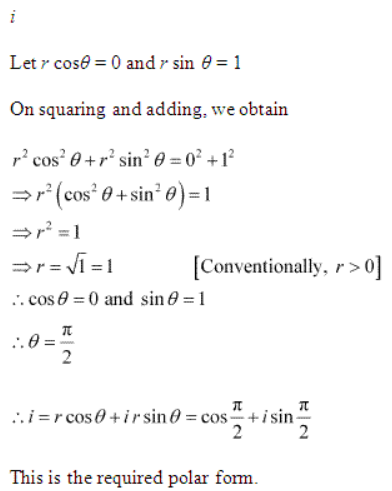### Solve each of the following equations:

Question1. Solve the equation x2 + 3 = 0

Solution :

General form  ax2+bx+c=0

We obtain  a=1,b=0,  and   c=3

Therefore, the discriminant of the given equation is

D=b2−4ac=02−4× 1 × 3=-12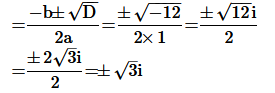Therefore, the required solutions are

Question2. Solve the equation 2x2 + x + 1 = 0

Solution :
Given: 2x2 + x + 1 = 0

General form  ax2+bx+c=0

We obtain  a=2,b=1,  and   c=1

Therefore, the discriminant of the given equation is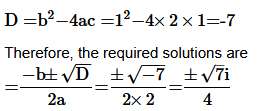Question3. Solve the equation x2 + 3x + 9 = 0

Solution :
Given: x2 + 3x + 9 = 0

General form  ax2+bx+c=0

We obtain  a=1,b=3,  and   c=9

Therefore, the discriminant of the given equation is

D=b2−4ac=32−4× 1 × 9=-27

Therefore, the required solutions are

=−b ± √D / 2a=−3± −√27 / 2 × 1=−3± 3√3i / 2

Question4. Solve the equation –x2 + x – 2 = 0

Solution :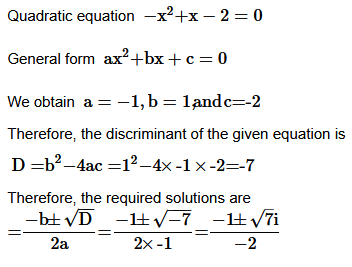Question5. Solve the equation x2 + 3x + 5 = 0

Solution :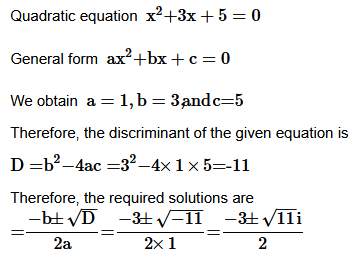Question6. Solve the equation x2 – x + 2 = 0

Solution :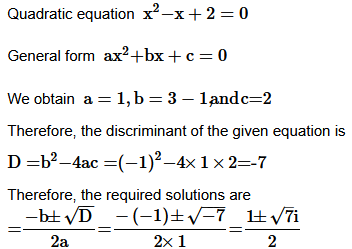Question7. √2x2 + x + √2 =0

Solution :
Given: √2x2 + x + √2 =0

Question8. √3x2 − √2x + 3√3 =0

Solution :
Given: √3x− √2x + 3√3 =0

Question9. x2 + x +1/√2 = 0

Solution :
Given: x2 + x +1/√2 = 0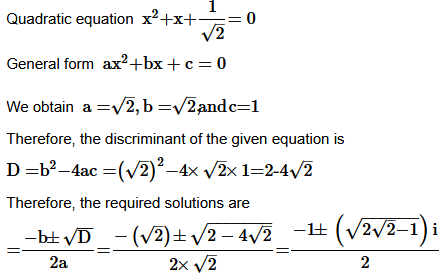,

Question10. x2 + x/√2 + 1 =0

Solution :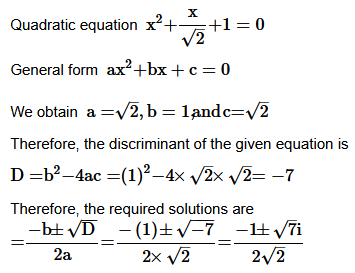Miscellaneous Exercise

Question1. Evaluate: [i18 + (1 / i)25 ]3

Solution :
Given: [i18 + (1 / i)25 ]3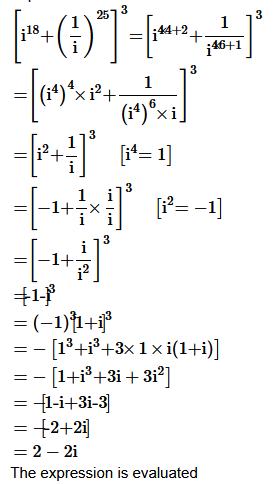Question2. For any two complex numbers z1 and z2, prove that
Re (z1z2)  =  Re z1  Re z2  –  Im z1 Im z2

Solution :
Let z1 = x1+  iy1  and z2 = x+ iy2
∴z1z= (x1+iy1) (x+ iy2)
=x1(x2 + iy2)  +  iy1(x2 + ty2)
=x1x +  ix1y2  +  iy1x2  +  i2y1y2
=x1x2 + ix1y2 + iy1x2 − y1y2
=(x1x2  −  y1y2) +  i(x2y2  +  y1x2)
⇒Re(zz2)=  x1x −  y1y2
⇒Re (zz2)  =  Rez1 Rez2  −  lmzImz2
Hence, proved.
Question3. Reduceto the standard form.

Solution :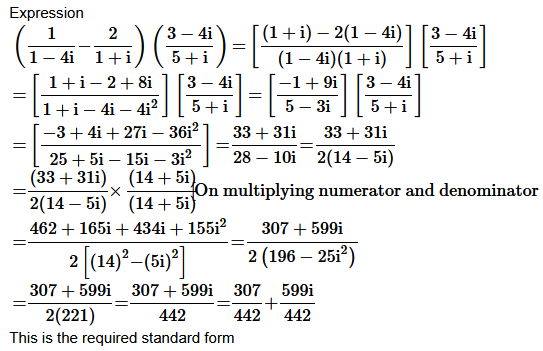Question4. If prove that

Solution :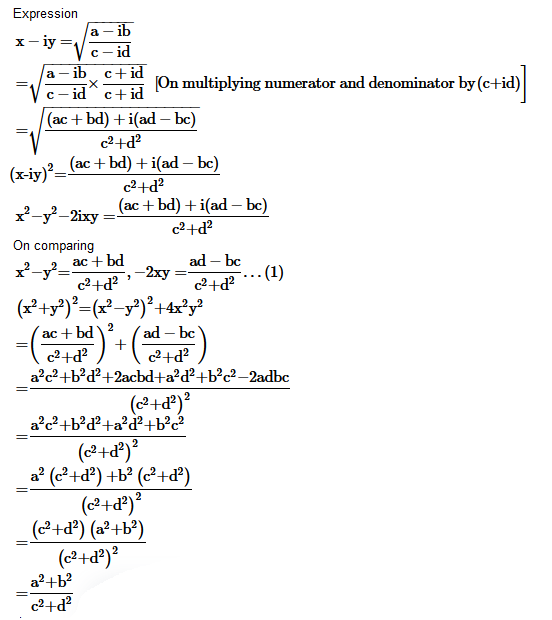Question5. Convert the following in the polar form:
(i)
(ii)

Solution :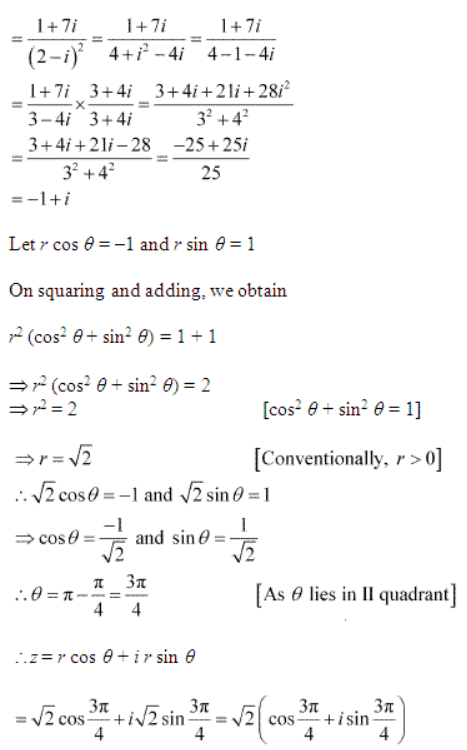This is the required polar form.

(ii) Here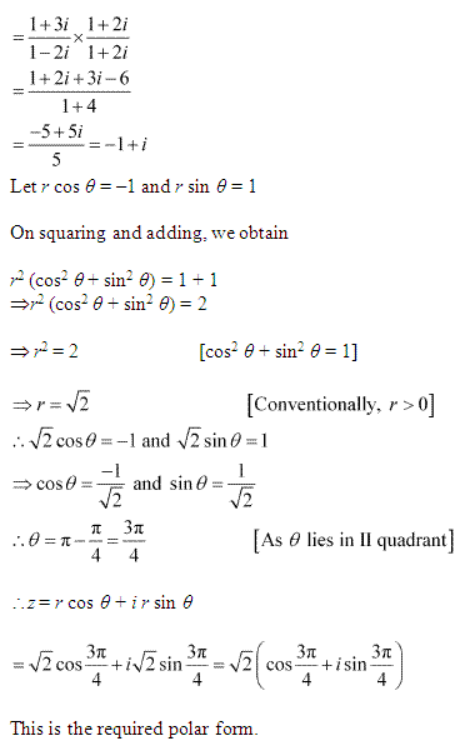Solve each of the equations in exercises 6 to 9:
Question6. 3x2 -4x + 20/3 = 0

Solution :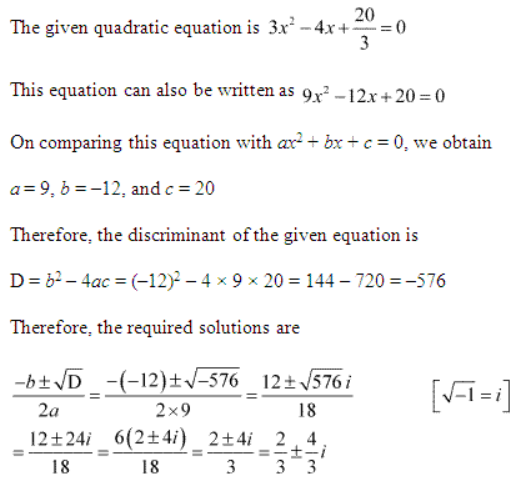Question7.  x2 -2x + 3/2 = 0

Solution. Given:

Question8. Solve the equation 27x– 10x + 1 = 0

Solution :
Given:

Question9. Solve the equation 21x– 28x + 10 = 0

Solution :
Given: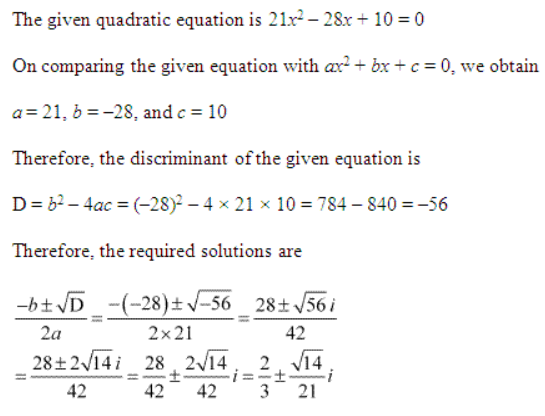Question10. If find

Solution :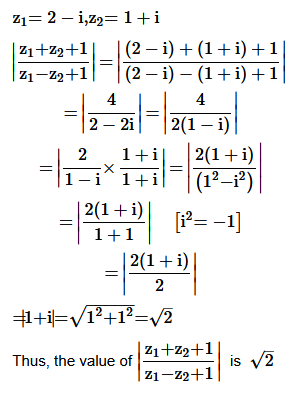Question11. If prove that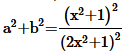Solution :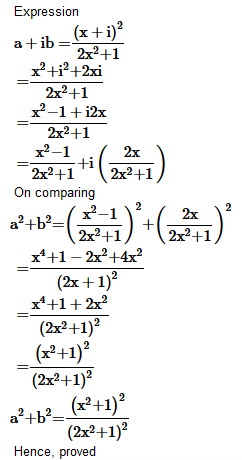Question12. Let z1=2−i,z2 =−2+i. Find
(i) Re (z1z2/z1),
(ii) Im (1z1/ z1)

Solution :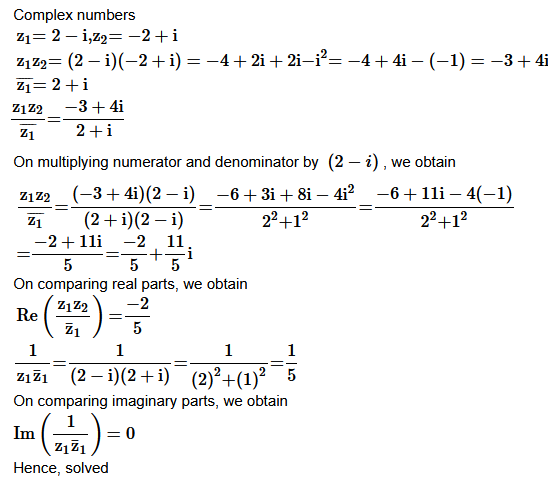Question13. Find the modulus and argument of the complex number

Solution :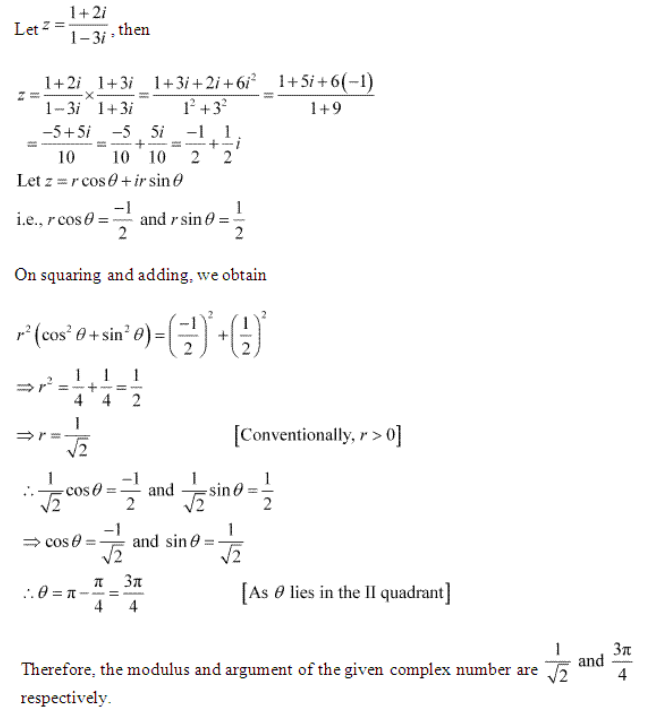Question14. Find the real numbers x and y if (x – iy) (3 + 5i) is the conjugate of –6 – 24i.

Solution :
Let = (x−ty) (3+5i)z
=3x + 5xi − 3yi − 5yi2
=3x + 5xi − 3yi + 5y
=(3x+5y) + i(5x−3y)
∴z =(3x+5y) −i (5x−3y)
It is given that,z=−6−24i
∴(3x+5y) −i(5x−3y) =−6−24i
Equating real and imaginary parts, we obtain
3x+5y=−6
5x−3y=24
Multiplying equation (i) by 3 and equation (ii) by 5 and then adding them, we obtain
Putting the value ofxin equation (i),
we obtain 3(3)+ 5y=−6⇒ 5y=−6−15 ⇒y=−3
Thus, the values of x and y are 3 and − 3 respectlvely.
Question15. Find the modulus of

Solution :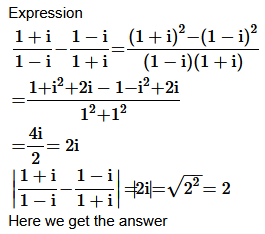Question16. If then show that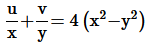Ans. Given: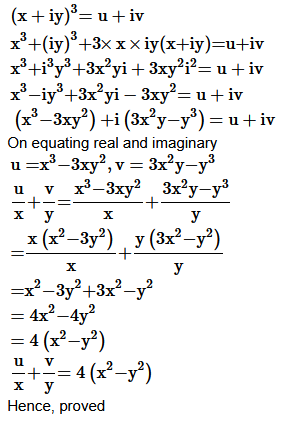Question17. If  α and  β are different complex numbers with | β | = 1  then find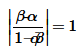Solution :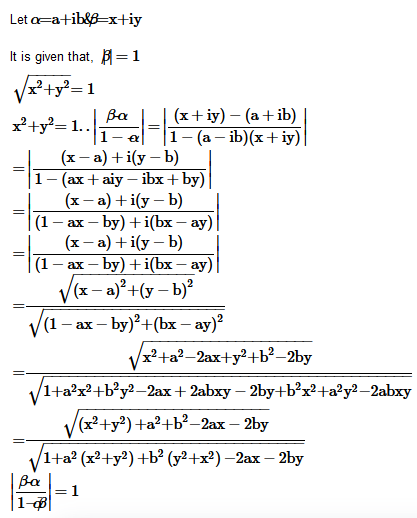Question 18. Find the number of non-zero integral solutions of the equation |1−i|= 2x

Solution :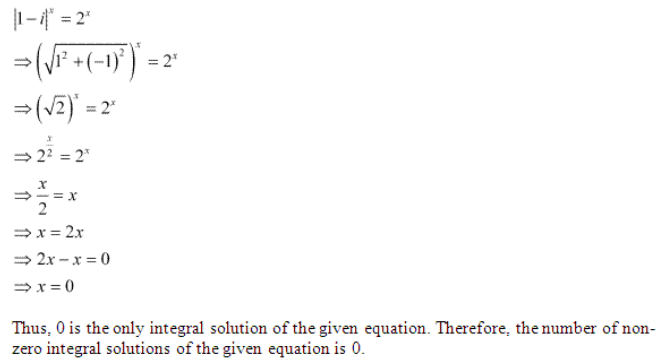Question19. If (a + ib) (c + id) (e + if) (g + ih) = A + iB, then show that
(a2 + b2) (c2 + d2) (e2 + f2) (g2 + h2) = A2 + B2.

Solution :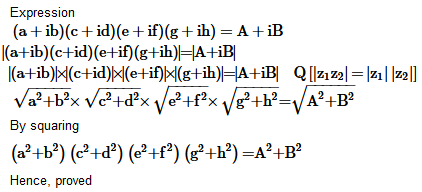Question20. If then find the least positive integral value of m.

Solution :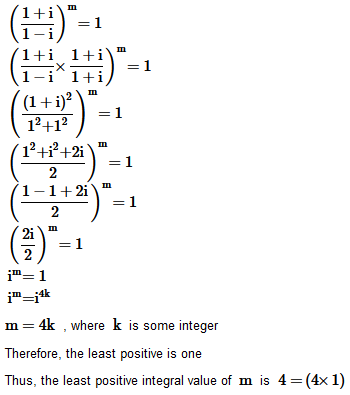Get 30% off your first purchase!

X
error: Content is protected !!
Scroll to Top